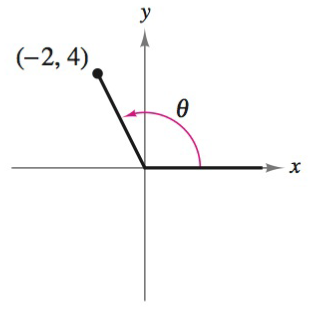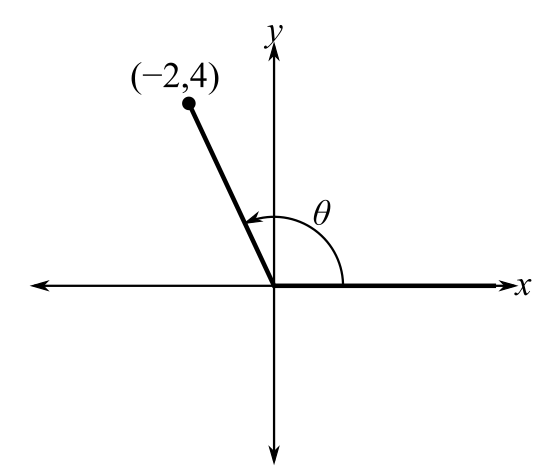Chapter 8.2, Problem 5E### Calculus: An Applied Approach (Min...

10th Edition
Ron Larson
ISBN: 9781305860919

#### Solutions

Chapter
Section### Calculus: An Applied Approach (Min...

10th Edition
Ron Larson
ISBN: 9781305860919
Textbook Problem
1 views

# Evaluating Trigonometric Functions In Exercises 1-6, find the exact values of the six trigonometric functions of the angle θ. See Example 1.To determine

To calculate: The exact values of the six trigonometric functions of the angle θ for the provided figure.Explanation

Given Information:

The figure provided below shows the angle θ.

Formula used:

The trigonometric functions are,

sinθ=yr         cscθ=rycosθ=xr         secθ=rxtanθ=yx         cotθ=xy

Where, x is the length of the side adjacent to θ, y is the length of the side opposite to θ, and r=x2+y2 is the length of hypotenuse r0.

Calculation:

From the provided figure, the value of x is 2 and y is 4.

Substitute x=2 and y=4 in the equation r=x2+y2.

r=(2)2+(4)2=4+16=20=25

Substitute y=4 and r=25 in sinθ=yr.

sinθ=425=425×55=255

Substitute x=2 and r=25 in cosθ=xr

### Still sussing out bartleby?

Check out a sample textbook solution.

See a sample solution

#### The Solution to Your Study Problems

Bartleby provides explanations to thousands of textbook problems written by our experts, many with advanced degrees!

Get Started

#### Find more solutions based on key concepts